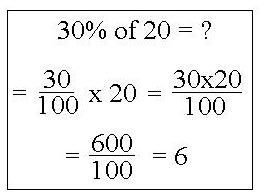# Harlan Bengtson## Four-Step Math Problem Solving Strategies & Techniques

Using one of more of the math problem solving strategies presented in this article can aid in solving your math problems. The problem solving techniques discussed here start with a four step procedure. Several useful techniques are given for each of the first two important steps.## Calculating Math Percentages, Including Example Percentage Calculations

In teaching about calculating math percentages, you can break the lesson down into several types of percentage calculations: calculating a percentage of a number; finding the percentage that one number is of another number; and how to calculate percentages from fractions and decimals.